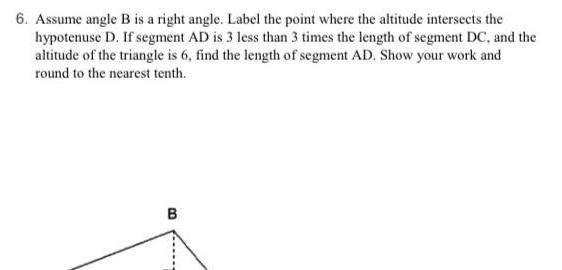Question:

# 6 Assume angle B is a right angle Label the point where the

Last updated: 8/7/20236 Assume angle B is a right angle Label the point where the altitude intersects the hypotenuse D If segment AD is 3 less than 3 times the length of segment DC and the altitude of the triangle is 6 find the length of segment AD Show your work and round to the nearest tenth B